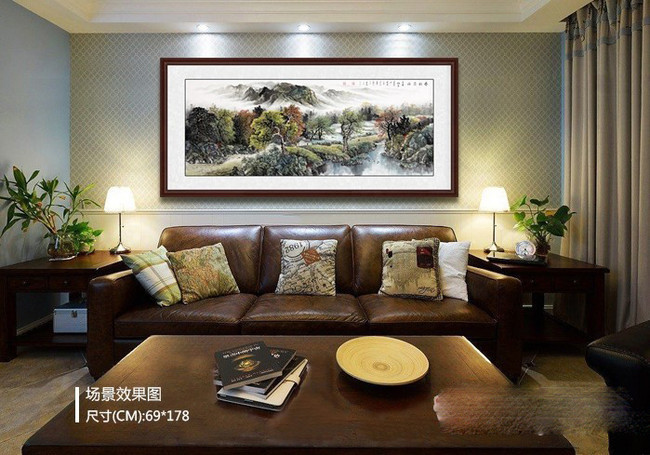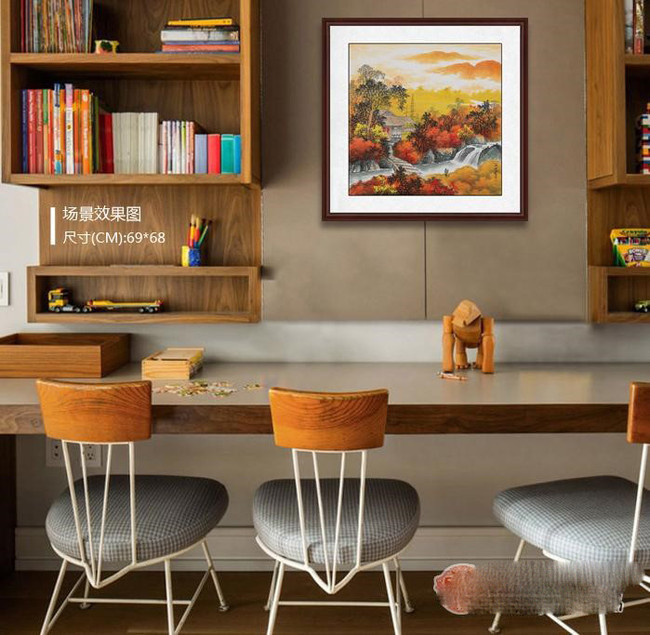|

# 客厅软装:山水画挂家里哪个位置比较好

客厅装饰画更多人都是青睐于山水画的，现代客厅挂画中，都少不了几幅山水画做点缀，但怎么挂得好看是有技巧的，有规律可行的。

对于居家风水来说，更加是个重点课题!山水画挂家里哪个位置比较好呢?怎么找到合适的位置?然后按什么样的悬挂法?没有主意的要仔细看咯!这四大黄金点一定要知道!

(一)、客厅沙发背后

沙发背后的墙面通常是客厅里最开阔的，适合布置一面挂画墙，会让单调的空间一下子成为亮点。从风水角度看，通常都是在沙发后面的墙上挂一幅风水山水画，与沙发形成山水相依的格局。水代表财源，流动的瀑布寓意财源滚滚，是非常好的，山代表靠山，背靠大山，不管做什么事都会有靠山，因此在客厅沙发后面挂山水画是非常理想的。

推荐一、沙发墙挂画 薛大庸最新六尺横幅山水画《春到深林》作品来源：易从网“疏林茅舍伴琴泉，云裳风诗卧清山。君姜自觉身心累，此处即是桃花源。”

此图绘巍峨雄伟群山，树木葱郁成林，山脚下平坦处，楼阁屋字错落有致，用笔工整细秀。此图山峦层叠，草木丰滋，山腰云雾环绕，山间溪水流淌，境界幽静清爽。其构图严谨，枯笔短皴，笔法浑厚，敷色润泽。是幅不可多得的佳作极具投资收藏价值，可悬挂在书房、客厅、会所、办公室等地。

推荐二、人气画家王宁最新力作聚宝盆作品《一帆风顺》其作品苍浑渊穆，气势磅礴，座座山峰堆叠而起，意境绵延悠长，烟云缥缈，掩映于山峰之间，气势磅礴的瀑布飞流直下，林木葱郁，其间点缀着小房子，生机勃勃。

欣赏此幅山水画作，可以感受到大自然的清新气息，素净典雅也不失活泼生动，挂在客厅有大山大水的雄伟壮阔，又有湖泊江水的动静之美，清新隽永耐人寻味，江流上船挂着满帆顺风行驶。包含着事事顺利，好运常来的美好愿望，此幅作品寓意极好，建议挂在客厅、酒店等地。

(二)、走廊玄关

狭长的走廊里，墙壁上选用了竖幅的装饰画来做点缀，色系相近，交错搭配，视觉空间无限延展。整个走廊仿佛艺术画廊，狭长的过道也因此变得耐看精巧。

推荐一、王宁竖幅山水画作品《溪山访友图》作品来源：易从网此幅《溪山访友图》用笔设色精巧细致，疏淡清润。笔墨浓重，意境清远，画法严谨古朴，师古人而创新，进一步发挥了笔墨清润的特点，气韵生动，骨法用笔，是幅不可多得的佳作，具有很高的艺术价值，极具投资收藏价值。将此画悬挂在茶楼、、玄关走道、酒店、会所彰显艺术品位及修养，作为礼品大气上档次。

推荐二、斗方装饰画搭配 王宁山水画作品《渔舟唱晚》作品来源：易从网一张组合柜，加一方地毯，构建了这个狭长过道里的休憩小空间。而紧贴着墙壁的斗方风景山水画，既不占空间，又足够舒适，给家平添一个精彩角落。

王宁的此幅斗方山水画，他挥去了透视中的景物排列，在笔墨之中，似又不拘笔墨苑囿;在山水之间，似又超乎山水之外。它是一种介乎于“出世”与“入世”之间的世界，是遥远的桃源仙境与平朴的田园牧歌相融合的世界。

(三)、餐厅

这个空挡作为装饰墙很合适，而且没什么禁忌。山水画中的斗方山水画，小巧精致，韵味十足，设色典雅，为整幅画面增添别致的韵味，观之流连忘返，品之心情舒畅，乃餐厅挂画的不错选择。

推荐、客厅餐厅风景画 王宁斗方山水画作品《秋韵》作品来源：易从网红枫叶如炬，秦岭起霓虹。任凭风落木，片叶颤秋浓。秋韵，醉上心头。画中景物层迭、树木茂密，巧妙地运用虚实、红黄的均衡布局，通过水的空灵和云雾的蒸腾，避免了过分的迫塞。呈现出深沉的秋意，高远之处晨雾迷离中若隐若现的茅屋，山涧下溪细细流淌，观此画可让人置身于幽美静谧的佳景之中。山主人丁水主财，王宁的此幅山水画作品《秋韵》作为风水画可悬挂在客厅餐厅，溪水长流寓意财源滚滚，事业长青。雅俗共赏的佳作也!

(四)、矮柜上

矮柜上方也有一块可发挥的空间，而且这个位置可适当搭配绿植、台灯、艺术摆件等，能与挂画组合得更有艺术感。

推荐、风景壁画 王宁斗方山水画作品《秋色人家》此幅《秋色人家》山水画笔墨爽利，清新秀逸，极富个性。作品线条流畅凝重，松柔秀拙，密密麻麻，在秋风吹拂下轻轻摇摆，呈一派辉煌绚烂之后重归静寂的大美景象。

欣赏王宁的这幅《秋色人家》山水画作品，苍茫深邃，清寂幽深，山岭逶迤不绝，密林生机盎然，瀑布如飞流而下，激荡魂魄，使观赏者身在画外，心在其中，心悦诚服的接受大自然美景的洗礼，闪耀着灿烂的异彩，挂在客厅彰显品位，提高档次，具另一番高雅;挂在办公室陶冶情操，放松心情，缓解压力，创新一流辉煌!

山水画挂在家里好不好?山水画挂家里哪个位置比较好?在了解了以上几条注意事项后，就可以根据这些内容来挑选合适合理的字画!易从网有很多山水画供您选择，还有专业的人员根据您的情况帮您挑选最合适的字画!

`声明：本文由入驻焦点开放平台的作者撰写，除焦点官方账号外，观点仅代表作者本人，不代表焦点立场错误信息举报电话： 400-099-0099，邮箱：jubao@vip.sohu.com，或点此进行意见反馈，或点此进行举报投诉。`A B C D E F G H J K L M N P Q R S T W X Y Z
A - B - C - D - E
• A
• 鞍山
• 安庆
• 安阳
• 安顺
• 安康
• 澳门
• B
• 北京
• 保定
• 包头
• 巴彦淖尔
• 本溪
• 蚌埠
• 亳州
• 滨州
• 北海
• 百色
• 巴中
• 毕节
• 保山
• 宝鸡
• 白银
• 巴州
• C
• 承德
• 沧州
• 长治
• 赤峰
• 朝阳
• 长春
• 常州
• 滁州
• 池州
• 长沙
• 常德
• 郴州
• 潮州
• 崇左
• 重庆
• 成都
• 楚雄
• 昌都
• 慈溪
• 常熟
• D
• 大同
• 大连
• 丹东
• 大庆
• 东营
• 德州
• 东莞
• 德阳
• 达州
• 大理
• 德宏
• 定西
• 儋州
• 东平
• E
• 鄂尔多斯
• 鄂州
• 恩施
F - G - H - I - J
• F
• 抚顺
• 阜新
• 阜阳
• 福州
• 抚州
• 佛山
• 防城港
• G
• 赣州
• 广州
• 桂林
• 贵港
• 广元
• 广安
• 贵阳
• 固原
• H
• 邯郸
• 衡水
• 呼和浩特
• 呼伦贝尔
• 葫芦岛
• 哈尔滨
• 黑河
• 淮安
• 杭州
• 湖州
• 合肥
• 淮南
• 淮北
• 黄山
• 菏泽
• 鹤壁
• 黄石
• 黄冈
• 衡阳
• 怀化
• 惠州
• 河源
• 贺州
• 河池
• 海口
• 红河
• 汉中
• 海东
• 怀来
• I
• J
• 晋中
• 锦州
• 吉林
• 鸡西
• 佳木斯
• 嘉兴
• 金华
• 景德镇
• 九江
• 吉安
• 济南
• 济宁
• 焦作
• 荆门
• 荆州
• 江门
• 揭阳
• 金昌
• 酒泉
• 嘉峪关
K - L - M - N - P
• K
• 开封
• 昆明
• 昆山
• L
• 廊坊
• 临汾
• 辽阳
• 连云港
• 丽水
• 六安
• 龙岩
• 莱芜
• 临沂
• 聊城
• 洛阳
• 漯河
• 娄底
• 柳州
• 来宾
• 泸州
• 乐山
• 六盘水
• 丽江
• 临沧
• 拉萨
• 林芝
• 兰州
• 陇南
• M
• 牡丹江
• 马鞍山
• 茂名
• 梅州
• 绵阳
• 眉山
• N
• 南京
• 南通
• 宁波
• 南平
• 宁德
• 南昌
• 南阳
• 南宁
• 内江
• 南充
• P
• 盘锦
• 莆田
• 平顶山
• 濮阳
• 攀枝花
• 普洱
• 平凉
Q - R - S - T - W
• Q
• 秦皇岛
• 齐齐哈尔
• 衢州
• 泉州
• 青岛
• 清远
• 钦州
• 黔南
• 曲靖
• 庆阳
• R
• 日照
• 日喀则
• S
• 石家庄
• 沈阳
• 双鸭山
• 绥化
• 上海
• 苏州
• 宿迁
• 绍兴
• 宿州
• 三明
• 上饶
• 三门峡
• 商丘
• 十堰
• 随州
• 邵阳
• 韶关
• 深圳
• 汕头
• 汕尾
• 三亚
• 三沙
• 遂宁
• 山南
• 商洛
• 石嘴山
• T
• 天津
• 唐山
• 太原
• 通辽
• 铁岭
• 泰州
• 台州
• 铜陵
• 泰安
• 铜仁
• 铜川
• 天水
• 天门
• W
• 乌海
• 乌兰察布
• 无锡
• 温州
• 芜湖
• 潍坊
• 威海
• 武汉
• 梧州
• 渭南
• 武威
• 吴忠
• 乌鲁木齐
X - Y - Z
• X
• 邢台
• 徐州
• 宣城
• 厦门
• 新乡
• 许昌
• 信阳
• 襄阳
• 孝感
• 咸宁
• 湘潭
• 湘西
• 西双版纳
• 西安
• 咸阳
• 西宁
• 仙桃
• 西昌
• Y
• 运城
• 营口
• 盐城
• 扬州
• 鹰潭
• 宜春
• 烟台
• 宜昌
• 岳阳
• 益阳
• 永州
• 阳江
• 云浮
• 玉林
• 宜宾
• 雅安
• 玉溪
• 延安
• 榆林
• 银川
• Z
• 张家口
• 镇江
• 舟山
• 漳州
• 淄博
• 枣庄
• 郑州
• 周口
• 驻马店
• 株洲
• 张家界
• 珠海
• 湛江
• 肇庆
• 中山
• 自贡
• 资阳
• 遵义
• 昭通
• 张掖
• 中卫

1室1厅1厨1卫1阳台

1
2
3
4
5

0
1
2

1

1

0
1
2
3报名成功，资料已提交审核A B C D E F G H J K L M N P Q R S T W X Y Z
A - B - C - D - E
• A
• 鞍山
• 安庆
• 安阳
• 安顺
• 安康
• 澳门
• B
• 北京
• 保定
• 包头
• 巴彦淖尔
• 本溪
• 蚌埠
• 亳州
• 滨州
• 北海
• 百色
• 巴中
• 毕节
• 保山
• 宝鸡
• 白银
• 巴州
• C
• 承德
• 沧州
• 长治
• 赤峰
• 朝阳
• 长春
• 常州
• 滁州
• 池州
• 长沙
• 常德
• 郴州
• 潮州
• 崇左
• 重庆
• 成都
• 楚雄
• 昌都
• 慈溪
• 常熟
• D
• 大同
• 大连
• 丹东
• 大庆
• 东营
• 德州
• 东莞
• 德阳
• 达州
• 大理
• 德宏
• 定西
• 儋州
• 东平
• E
• 鄂尔多斯
• 鄂州
• 恩施
F - G - H - I - J
• F
• 抚顺
• 阜新
• 阜阳
• 福州
• 抚州
• 佛山
• 防城港
• G
• 赣州
• 广州
• 桂林
• 贵港
• 广元
• 广安
• 贵阳
• 固原
• H
• 邯郸
• 衡水
• 呼和浩特
• 呼伦贝尔
• 葫芦岛
• 哈尔滨
• 黑河
• 淮安
• 杭州
• 湖州
• 合肥
• 淮南
• 淮北
• 黄山
• 菏泽
• 鹤壁
• 黄石
• 黄冈
• 衡阳
• 怀化
• 惠州
• 河源
• 贺州
• 河池
• 海口
• 红河
• 汉中
• 海东
• 怀来
• I
• J
• 晋中
• 锦州
• 吉林
• 鸡西
• 佳木斯
• 嘉兴
• 金华
• 景德镇
• 九江
• 吉安
• 济南
• 济宁
• 焦作
• 荆门
• 荆州
• 江门
• 揭阳
• 金昌
• 酒泉
• 嘉峪关
K - L - M - N - P
• K
• 开封
• 昆明
• 昆山
• L
• 廊坊
• 临汾
• 辽阳
• 连云港
• 丽水
• 六安
• 龙岩
• 莱芜
• 临沂
• 聊城
• 洛阳
• 漯河
• 娄底
• 柳州
• 来宾
• 泸州
• 乐山
• 六盘水
• 丽江
• 临沧
• 拉萨
• 林芝
• 兰州
• 陇南
• M
• 牡丹江
• 马鞍山
• 茂名
• 梅州
• 绵阳
• 眉山
• N
• 南京
• 南通
• 宁波
• 南平
• 宁德
• 南昌
• 南阳
• 南宁
• 内江
• 南充
• P
• 盘锦
• 莆田
• 平顶山
• 濮阳
• 攀枝花
• 普洱
• 平凉
Q - R - S - T - W
• Q
• 秦皇岛
• 齐齐哈尔
• 衢州
• 泉州
• 青岛
• 清远
• 钦州
• 黔南
• 曲靖
• 庆阳
• R
• 日照
• 日喀则
• S
• 石家庄
• 沈阳
• 双鸭山
• 绥化
• 上海
• 苏州
• 宿迁
• 绍兴
• 宿州
• 三明
• 上饶
• 三门峡
• 商丘
• 十堰
• 随州
• 邵阳
• 韶关
• 深圳
• 汕头
• 汕尾
• 三亚
• 三沙
• 遂宁
• 山南
• 商洛
• 石嘴山
• T
• 天津
• 唐山
• 太原
• 通辽
• 铁岭
• 泰州
• 台州
• 铜陵
• 泰安
• 铜仁
• 铜川
• 天水
• 天门
• W
• 乌海
• 乌兰察布
• 无锡
• 温州
• 芜湖
• 潍坊
• 威海
• 武汉
• 梧州
• 渭南
• 武威
• 吴忠
• 乌鲁木齐
X - Y - Z
• X
• 邢台
• 徐州
• 宣城
• 厦门
• 新乡
• 许昌
• 信阳
• 襄阳
• 孝感
• 咸宁
• 湘潭
• 湘西
• 西双版纳
• 西安
• 咸阳
• 西宁
• 仙桃
• 西昌
• Y
• 运城
• 营口
• 盐城
• 扬州
• 鹰潭
• 宜春
• 烟台
• 宜昌
• 岳阳
• 益阳
• 永州
• 阳江
• 云浮
• 玉林
• 宜宾
• 雅安
• 玉溪
• 延安
• 榆林
• 银川
• Z
• 张家口
• 镇江
• 舟山
• 漳州
• 淄博
• 枣庄
• 郑州
• 周口
• 驻马店
• 株洲
• 张家界
• 珠海
• 湛江
• 肇庆
• 中山
• 自贡
• 资阳
• 遵义
• 昭通
• 张掖
• 中卫• 手机• 分享
• 设计
免费设计
• 计算器
装修计算器
• 入驻
合作入驻
• 联系
联系我们
• 置顶
返回顶部